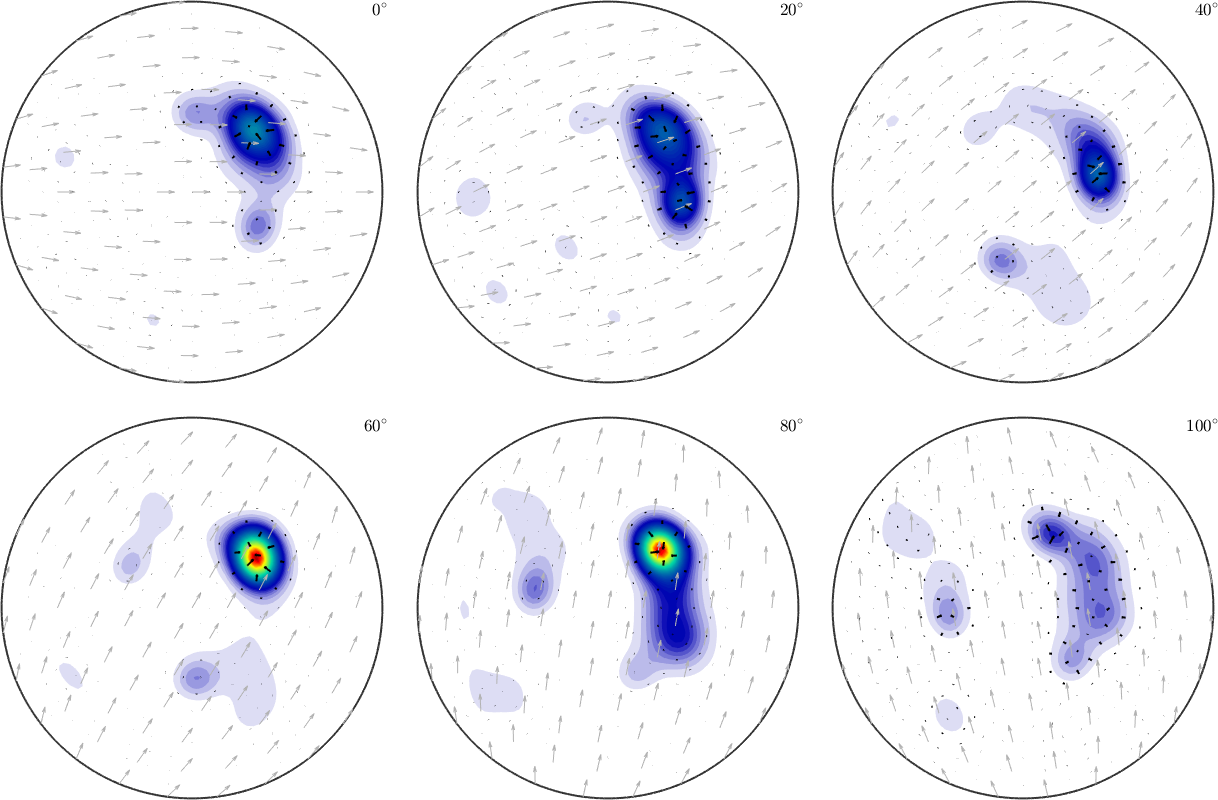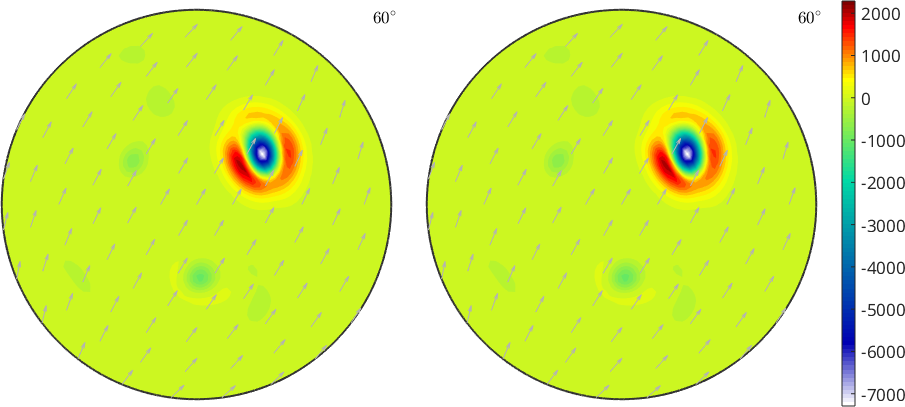SO3VectorField edit page

SO3VectorField handles three-dimensional functions on the rotation group. For instance the gradient of an univariate SO3FunHarmonic can return a SO3VectorFieldHarmonic.

## Defining a SO3VectorFieldHandle

Analogous to SO3FunHandle we are able to define SO3VectorFields by an anonymous function.

Note that the evaluations are of class vector3d

## Defining a SO3VectorFieldHarmonic

Definition via SO3VectorField

We can expand any SO3VectorField in an SO3VectorFieldHarmonic directly by the command SO3VectorFieldHarmonic

Definition via function values

At first we need some example rotations

Next, we define function values for the rotations

Now the actual command to get SO3VF1 of type SO3VectorFieldHarmonic

Definition via function handle

If we have a function handle for the function we could create a S2VectorFieldHarmonic via quadrature. At first lets define a function handle which takes rotation as an argument and returns a vector3d:

Now we can call the quadrature command to get SO3VF2 of type SO3VectorFieldHarmonic

Definition via SO3FunHarmonic

If we directly call the constructor with a multivariate SO3FunHarmonic with three entries it will create a SO3VectorFieldHarmonic with SO3F(1), SO3F(2), and SO3F(3) the $$x$$, $$y$$, and $$z$$ component.

## Operations

Basic arithmetic operations

Again the basic mathematical operations are supported:

addition/subtraction of a vector field and a vector or addition/subtraction of two vector fields

multiplication/division by a scalar or a SO3Fun

dot product with a vector or another vector field

cross product with a vector or another vector field

mean vector of the vector field

rotation of the vector field

pointwise norm of the vectors

## Visualization

One can use the default plot-command• same as quiver(sVF1)

or the 3D plot of the rotation group with the vectors on itself